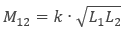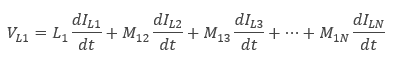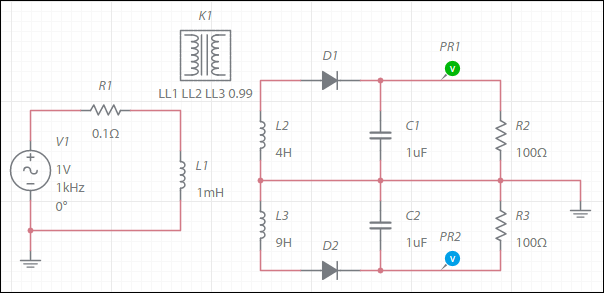OK

# Inductor coupling

Use this component to introduce mutual inductance between inductors.

This component does not get wired into the circuit.

The mutual inductance between any two inductors, L1 and L2, is defined by:where k is the coupling coefficient.

Thus, in a set of inductors L1,L2,L3…LN, the voltage across inductor L1 is defined by:Example